# Hydraulic Power in Crude Oil Systems

The classic equation used in hydro power applications is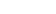where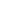is the power output,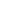is the flow rate,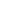is the available hydraulic head across the machine, andis acceleration due to gravity. This equation assumes the fluid is water. Specific gravity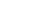is therefore omitted for clarity.  Note that this equation is applicable to pump or turbine configurations as they are the same machine. In crude oil service where the density is not equal to that of water,is included: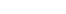whereis the fluid density. A common adaptation of these equations is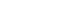whereis the power output in horsepower (convenient for pump prime mover sizing),is flow rate in gallons per minute andis the specific gravity. Expressingin kW (convenient for turbine electrical power output calculations) yields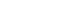These equations do not account for inefficiencies of the energy conversion process. The power available to recover from a hydraulic power recovery turbine in crude oil service accounting for fluid density, turbine and generator inefficiencies is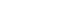whereis the power output in kW,is flow rate in gallons per minute,is the differential hydraulic head in feet,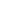is turbine efficiency,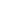is the generator efficiency andis the specific gravity of the fluid.

## References

 H. Addison, A Treatise on Applied Hydraulics, Fifth. London: Chapman and Hall Limited, 1964.

Note: This is a revised, WordPress friendly adaptation of content from Brendon Bruns’ Petroleum Engineering Masters project: https://scholarworks.alaska.edu/handle/11122/10947